# Multiplication Worksheets for Beginners

Multiplication worksheets for beginners are exclusively available on this page. There are various exciting exercises like picture multiplication, repeated addition, missing factors, comparing quantities, forming the products and lots more! These pdf worksheets are recommended for 2nd grade through 5th grade. Kick into practice with the free worksheets here!

## Picture Multiplication

Multiplication models: Exclusive

This exclusive page has over 90 printable worksheets on multiplication models like writing multiplication sentences using equal groups, area, arrays and number lines.

Multiplication Models Worksheets

Multiplication using pictures

The kids may use the pictures to solve the multiplication problems. E.g., how many legs do 4 bugs have?Cost of the objects

Multiply the number of objects with their cost to find the total cost of the objects.Fill in the box with the correct multiplicand for each repeated addition and find the product. The easy level has factors from 2 to 5 and the moderate level has factors from 2 to 10.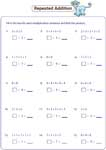Easy:

Moderate:

Rewrite each multiplication sentence into addition sentences in two possible ways.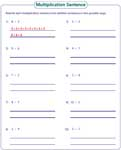## Multiplication Practice with Word Problems

Multiplication for beginners

These multiplication pdf worksheets are for beginners. The first three handouts have factors up to 10 and the next three have factors up to 12.Factors up to 10:

Factors up to 12:

2-digit multiplication

Each worksheet has 12 standard problems and 2 word problems.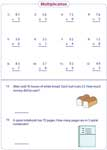2-digit by 1-digit:

2-digit by 2-digit:

Multiplication drills

There is a huge collection (over 160 worksheets) of multiplication drills on factors up to 10, factors up to 12, 2-digit times 1-digit and 2-digit times 2-digit.

Multiplication Drill Worksheets

The printer-friendly worksheets in this page have large number multiplicands like 4-digit, 5-digit, 6-digit and more. Real-life problems are also included.

Multiplying large numbers Worksheets

## Multiplying More Numbers

Multiplying three numbers

Each pdf worksheet has 15 problems multiplying three factors. The factors are arranged horizontally.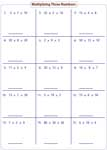Multiplying four numbers

There are four multiplicands in each problem. Multiply them and find the product.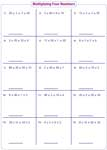## Missing Factors

Complete the multiplication sentence

Complete the multiplication sentences by filling in the box with the missing factors.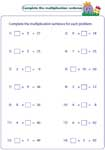Factors up to 10:

Factors up to 12:

Balancing the equation

The students should find the missing factor to balance the multiplication equation.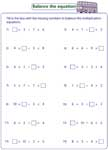Factors up to 10:

Factors up to 12:

## Comparing Quantities

Cut-paste activity

This cut and paste activity is based on comparing the quantities and identifying the greater one. Alligator and kingfisher themes are used in the easy and moderate level respectively.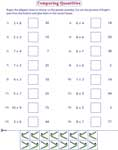Easy:

Moderate:

Identifying correct symbol

The printable worksheets here have 14 multiplication problems. Fill in the boxes with the correct symbols like <,> or = that satisfies each problem.Easy:

Moderate:

Selecting possible solutions

Each inequality in this worksheet has more than one solution. Select all possible choices that satisfy each inequality.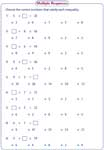Easy:

Moderate:

## Forming the products

Help the hen

The hen has a number (product) on it. The kids should help the hen to find the correct eggs that result in the product.Equivalent multiplication sentences

Identify the option(s) that is equivalent to each multiplication sentence in the worksheets.Related Worksheets